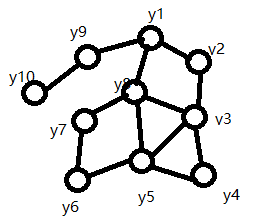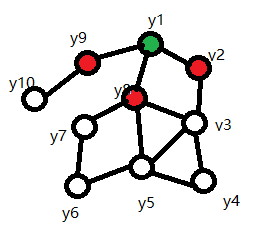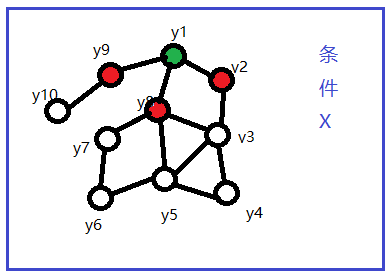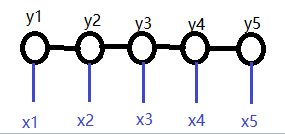# CRF(条件随机场)

## 基本概念

1. 场是什么
场就是一个联合概率分布。比如有3个变量，y1,y2,y3, 取值范围是{0,1}。联合概率分布就是{P(y2=0|y1=0,y3=0), P(y3=0|y1=0,y2=0), P(y2=0|y1=1,y3=0), P(y3=0|y1=1,y2=0), ...}
下图就是一个场的简单示意图。2. 马尔科夫随机场1. 条件随机场
有随机变量X(x1,x2,...), Y(y1,y2,...), 在给定X的条件下Y的概率分布是P(Y|X)。如果该分布满足马尔科夫性，即只和相邻变量有关，则称为条件随机场。
如下图，与马尔科夫随机场的区别是多了条件X。2. 线性链条件随机场
随机变量Y成线性，即每个变量只和前后变量相关。
当条件X与变量Y的形式相同时，就是如下图所示的线性链条件随机场。该形式也是最常使用的，广泛用于词性标注，命名实体识别等问题。## 线性链条件随机场的表示

$P(Y|X)$是线性链条件随机场，则在给定$X$的取值$x$的情况下，随机变量$Y$取值为$y$的条件概率可以表达为：

$P(y|x)=\frac{1}{Z(x)}exp\left(\sum_{i,k}{\lambda_kt_k(y_{i-1}, y_i,x,i)}+\sum_{i,l}\mu_ls_l(y_i,x,i)\right)$

$Z(x)=\sum_yexp\left(\sum_{i,k}{\lambda_kt_k(y_{i-1}, y_i,x,i)}+\sum_{i,l}\mu_ls_l(y_i,x,i)\right)$

$i$: 表示当前位置下标
$t_k()$：表示相邻两个输出间的关系，是转移特征函数。取值{0，1}，即满足特征和不满足特征
$s_l()$: 表示当前位置的特征，是状态特征函数。取值{0，1}。
$k$: 表示转移特征$t$的个数
$l$: 表示状态特征$s$的个数

## 条件随机场的化简形式和矩阵形式

#### 条件随机场的化简形式

$f_k(y_{i-1},y_i,x,i)=\left\{ \begin{array}{lcl} t_k(y_{i-1},y_i,x,i), & k=1,2,...K_1\\ s_l(y_i,x,i), & k=K_1+l; l=1,2,...K_2 \\ \end{array} \right.$

$f_k(y,x)=\sum_{i=1}^{n}f_k(y_{i-1},y_i,x,i),\quad k=1,2,...,K$

$\omega_k$表示特征$f_k(y,x)$的权值，即

$\omega_k=\left\{ \begin{array}{lcl} \lambda_k, & {k=1,2,...,K_1} \\ \mu_l, & {k=K_1+l; l=1,2,...,K_2} \end{array} \right.$

$P(y|x)=\frac{1}{Z(x)}exp\sum_{k=1}^{K}\omega_kf_k(y,x)$

$Z(x)=\sum_{y}exp\sum_{k=1}^{K}\omega_kf_k(y,x)$

$\omega$表示权值向量，即

$\omega=(\omega_1,\omega_2,...\omega_k)^{T}$

$F(y,x)$表示全局特征向量，即

$F(y,x)=(f_1(y,x),f_2(y,x),...,f_K(y,x))^{T}$

$P_\omega(y|x)=\frac{exp(\omega\cdot F(y,x))}{Z_\omega(x)}$

$Z_\omega(x)=\sum_{y}exp(\omega\cdot F(y,x))$

#### 条件随机场的矩阵形式

$y$一共有$m$种取值，则定义一个$m\times m$的矩阵

$M_i(x)=[M_i(y_{i-1},y_i|x)]$

$M_i(y_{i-1},y_i|x)=exp\left(W_i(y_{i-1},y_i|x)\right)$

$W_i(y_{i-1},y_i|x)=\sum_{k=1}^{K}\omega_kf_k(y_{i-1},y_i,x,i)$

$M_i(y_{i-1},y_i|x)$中的$y$取值是固定的，$M_i(x)$则是合并了所有可能的$y$取值，在矩阵表示下条件概率可以表达为$P_\omega(y|x)$

$P_\omega(y|x)=\frac{1}{Z_\omega(x)}\prod_{i=1}^{n+1}M_i(y_{i-1},y{i}|x)$

$Z_\omega(x)=(M_1(x)M_2(x)...M_{n+1}(x))_{start,stop}$

## CRF涉及的三个问题

1. 条件随机场的概率计算问题
2. 条件随机场的学习算法
3. 条件随机场的预测算法

## 条件随机场的概率计算问题

$P(Y_i=y_i|x)=\frac{\alpha_{i}^{T}(y_i|x)\beta_{i}^{T}(y_i|x)}{Z(x)}$

$P(Y_{i-1}=y_{i-1},Y_i=y_i|x)=\frac{\alpha_{i-1}^{T}(y_{i-1}|x)M_i(y_{i-1},y_i|x)\beta_{i}^{T}(y_i|x)}{Z(x)}$

## 条件随机场的学习算法

$\prod_{x,y}P_\omega(y|x)^{\tilde{P}(x,y)}$

$L(\omega)=log\prod_{x,y}P_\omega(y|x)^{\tilde{P}(x,y)}$

## 条件随机场的预测算法

$\max_y\sum_{i=1}^{n}\omega\cdot F_i(y_{i-1},y_{i},x)$

$F_i(y_{i-1},y_i,x)=(f_1(y_{i-1},y_i,x,i),f_2(y_{i-1},y_i,x,i),...,f_K(y_{i-1},y_i,x,i))^T$

$\delta_i(j)$,仅考虑从起始位置到到当前位置$i$这段序列,在位置$i$上，上面目标函数在$y=j$时取得的最大值
$\psi_i(l)=j$$i$表示当前位置，$l$表示当前位置$y_i$的取值，$j$是前一个位置$y_{i-1}$的取值。也就是记录最大值获取的路径。

$\delta_i(l)=\max_{1\leq j\leq m}\{\delta_{i-1}(j)+\omega\cdot F_i(y_{i-1}=j,y_i=l,x)\},\quad l=1,2,...,m$

$\psi_i(l)=arg\max_{1\leq j\leq m}\{\delta_{i-1}(j)+\omega\cdot F_i(y_{i-1}=j,y_i=l,x)\},\quad l=1,2,...,m$

## 参考资料

1. 李航《统计学习方法》
posted @ 2018-12-03 18:32  匡子语  阅读(1538)  评论(0编辑  收藏